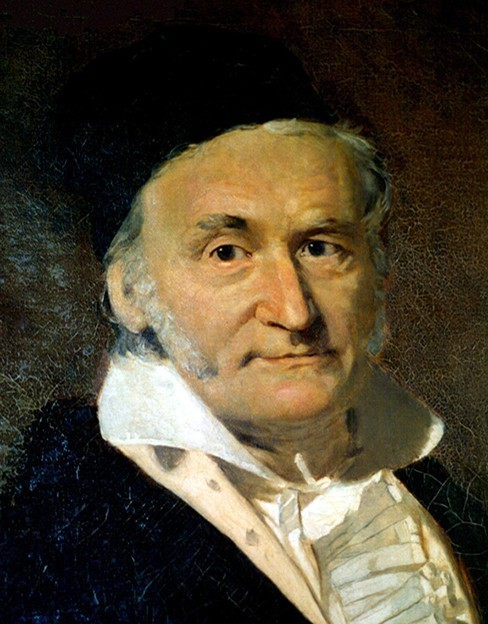## Introduction to Solving Systems with Gaussian Elimination

### Learning Objectives

By the end of this section, you will be able to:

• Write the augmented matrix of a system of equations.
• Write the system of equations from an augmented matrix.
• Perform row operations on a matrix.
• Solve a system of linear equations using matrices.Figure 1. German mathematician Carl Friedrich Gauss (1777–1855).

Carl Friedrich Gauss lived during the late 18th century and early 19th century, but he is still considered one of the most prolific mathematicians in history. His contributions to the science of mathematics and physics span fields such as algebra, number theory, analysis, differential geometry, astronomy, and optics, among others. His discoveries regarding matrix theory changed the way mathematicians have worked for the last two centuries.

We first encountered Gaussian elimination in Systems of Linear Equations: Two Variables. In this section, we will revisit this technique for solving systems, this time using matrices.